## 3.24Example of building a matrix equation

The previous sections describe methods to discretise derivative and other terms in order to build a matrix equation for a given physical equation. Let us demonstrate the construction of a matrix equation, using the momentum conservation equation from Sec. 3.23 as an example. It is a vector equation, so produces 3 matrix equations for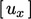,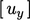and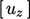.

The ﬁrst term, the time derivative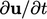, might be discretised with the Euler scheme Eq. (3.21 ). Matrix equations are constructed in extensive form as discussed in Sec. 3.6 . Hence, the contributions from Eq. (3.21 ) to matrix coeﬃcients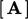and source vector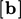are scaled by cell volume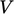, i.e.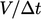and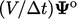, respectively, as illustrated below.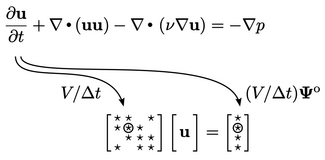The second term, the advective derivative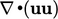, is discretised by Eq. (3.8 ). It pre-calculates the volumetric ﬂux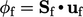, using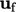interpolated by Eq. (3.3 ) with linear weights Eq. (3.4 ).

The transported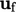might be discretised using the linear upwind scheme described in Sec. 3.14 . The scheme ﬁrst applies upwind discretisation, which contributes outgoing positive ﬂuxes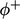to diagonal coeﬃcients and negative ﬂuxesto oﬀ-diagonals. It then adds an explicit contribution based on an extrapolated gradient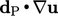(see Sec. 3.14 ). The gradient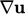is usually calculated by Eq. (3.18 ) with gradient limiting from Sec. 3.16 .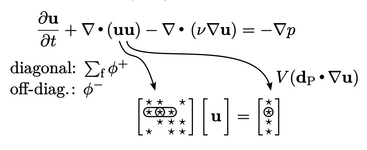The third term, the Laplacian derivative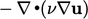, is discretised by Eq. (3.2 ). It requires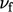, which is linearly interpolated from the cell centres. If the surface normal gradient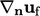includes a non-orthogonal correction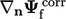, see Sec. 3.8 , then the term contributes to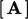and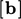, as shown below.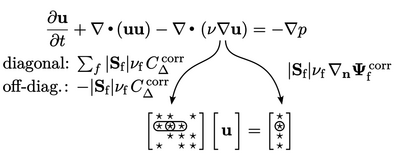The ﬁnal term,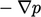is calculated using Eq. (3.18 ). Like all the other terms described here, it is implemented in extensive form, scaling by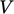, so is calculated for each cell by the vector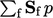. The relevant component (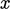,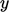,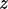) of this vector is then applied to the respective equation for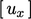,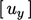and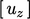.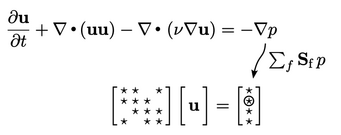Notes on CFD: General Principles - 3.24 Example of building a matrix equation